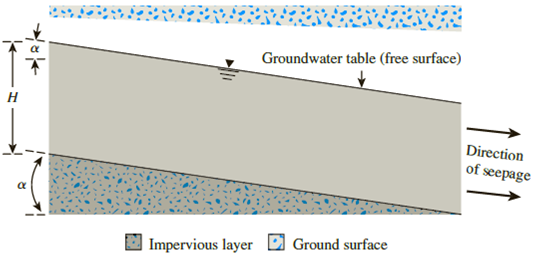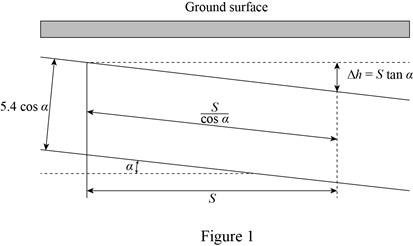# A permeable soil layer is underlain by an impervious layer as shown in Figure 7.29. Knowing that k = 6 × 10 −3 cm/sec for the permeable layer, calculate the rate of seepage through this layer in m 3 /hr/m width. Given: H = 5.4 m and α = 7°. Figure 7.29### Principles of Geotechnical Enginee...

9th Edition
Braja M. Das + 1 other
Publisher: Cengage Learning
ISBN: 9781305970939### Principles of Geotechnical Enginee...

9th Edition
Braja M. Das + 1 other
Publisher: Cengage Learning
ISBN: 9781305970939

#### Solutions

Chapter
Section
Chapter 7, Problem 7.1P
Textbook Problem

## A permeable soil layer is underlain by an impervious layer as shown in Figure 7.29. Knowing that k = 6 × 10−3 cm/sec for the permeable layer, calculate the rate of seepage through this layer in m3/hr/m width. Given: H = 5.4 m and α = 7°.Figure 7.29

Expert Solution
To determine

Find the rate of seepage through the permeable layer.

The rate of seepage through the permeable layer is 14.1×102m2/hr/m_.

### Explanation of Solution

Given information:

The hydraulic conductivity of the permeable soil layer k is 6×103cm/sec.

The height of the layer H is 5.4 m.

The angle value α is 7°.

Calculation:

Draw the free body diagram of the permeable layer as in Figure (1).Refer Figure (1).

Determine the hydraulic gradient using the relation.

i=ΔhL=StanαScosα=sinαcosα×cosα=sinα (1)

Here, Δh is the head loss and L is the horizontal length of the layer.

Substitute 7° for α in Equation (1).

i=sin7°=0.122

Determine the rate of seepage through the permeable layer using the relation.

q=kiA=ki(Hcosα×1)

Here, A is the area of the permeable layer.

Substitute 6×103cm/sec for k, 0.122 for i, 5.4 m for H, and 7° for α.

q=6×103cm/sec×(1m100m)×3,600sec1hr×0.122×5.4×cos7°×1=14.1×102m3/hr/m

Therefore, the rate of seepage through the permeable layer is 14.1×102m2/hr/m_.

### Want to see more full solutions like this?

Subscribe now to access step-by-step solutions to millions of textbook problems written by subject matter experts!

Get Solutions

### Want to see more full solutions like this?

Subscribe now to access step-by-step solutions to millions of textbook problems written by subject matter experts!

Get Solutions

Find more solutions based on key concepts
Show solutions
For Problems 16.1 through 16.19, draw the top, the front, and the right-side orthographic views of the objects ...

Engineering Fundamentals: An Introduction to Engineering (MindTap Course List)

Describe what is involved in feasibility analysis.

Fundamentals of Information Systems

What is an embedded system? Give three examples of such a system.

Principles of Information Systems (MindTap Course List)

Explain Appleâ€™s view of user interface design, especially for apps.

Systems Analysis and Design (Shelly Cashman Series) (MindTap Course List)

What is the range of addresses that might be assigned by APIPA?

Network+ Guide to Networks (MindTap Course List)

Which type of light is the most likely to cause burns? Why?

Welding: Principles and Applications (MindTap Course List)

Explain security concerns regarding BYOD policies.

Enhanced Discovering Computers 2017 (Shelly Cashman Series) (MindTap Course List)

If your motherboard supports ECC DDR3 memory, can you substitute non-ECC DDR3 memory?

A+ Guide to Hardware (Standalone Book) (MindTap Course List)